# Solver in ExcelArticle byExcelMojo Team## What Is Solver In Excel?

Solver in Excel is a special tool with flexible options and features to solve linear and non-linear programming problems. So, solver in excel is also called ‘Linear Programming Solver.’ Solver helps users change the values of multiple variables and provides desired data based on the specified criteria and conditions.

Before learning about solver, consider the below table that shows two variables, X and Y, in column A. The total of the two variables must be equal to 100. Also, the variables must satisfy the two conditions.

• The value of the X variable should be greater than or equal to 60 (>= 60)
• And, the value of the Y variable should be lesser than or equal to 30 (<=30)

So, we need to use the following steps to find the values of the two variables using solver in excel.

• First, type the SUM function in cell B4.
• Next, select the Solver option from the Analyze group.
• Finally, click OK to see the values of the two variables.

###### Key Takeaways
• Solver is a special Excel tool with many features used to solve linear and non-linear programming problems.
• The Solver in Excel is hidden by default. We can add it using Home -> Options -> Add-ins -> Solver Add-ins.
• One should first create a problem model to apply solver in Excel.
• The conditions in solver in excel can be greater than or equal to (>=), lesser than or equal to (<=), equal to (=).
• One should know statistical methods like GRG Nonlinear, Evolutionary, and Simplex LP concepts to apply solver in excel while solving related problems.

### How To Add Solver In Excel?

The Solver feature was introduced in Excel in the year 1991. Even though it is a built-in tool by default, it is always hidden.

The steps to enable or add Solver in Excel are as follows:

1. Go to the File tab.2. Click on Options.3. The Excel Options window appears.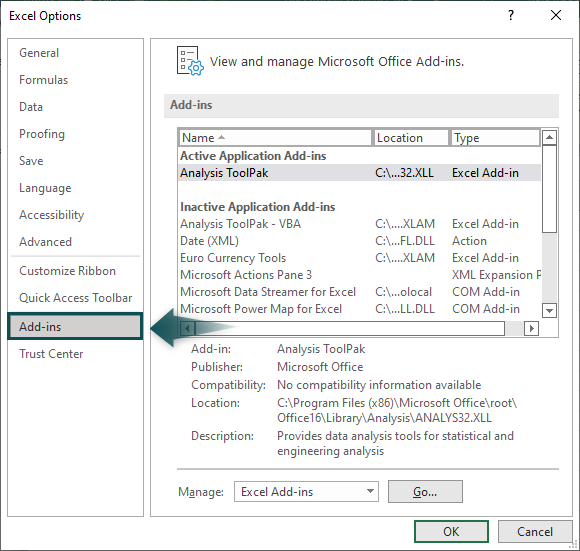5. Click on Go.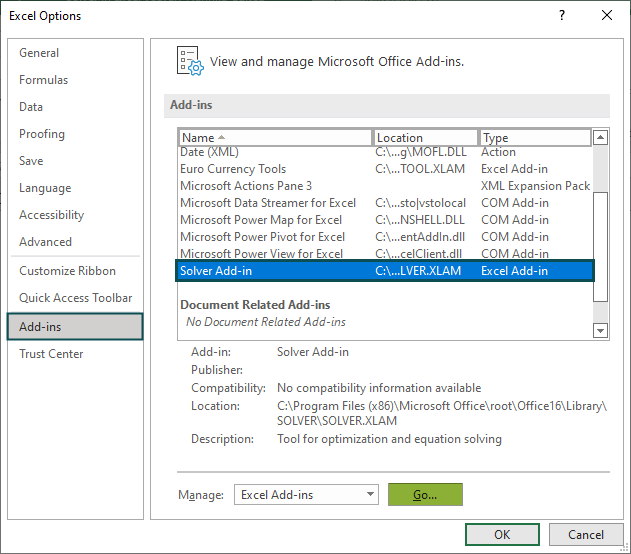6. The Add-ins window pops up.

7. Click OK.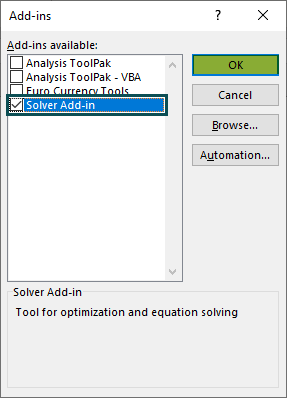8. We can see Solver option under the Analyze group in the Data tab.Similarly, we can enable or add solver in excel.

Please Note: After we click OK, excel may hang for 5 seconds. Meanwhile, the application takes this time to add Solver option under the Data tab.

### Excel Solver Terminologies

Let us understand the terminologies used while working with solver in excel with the following steps.

• Go to the Data tab.
• Choose the Solver option.
• The window, Solver Parameters appears.
##### Terminologies of Solver Parameter window

Let us learn all the six terminologies to use solver in excel function effectively.

Set Objective: We should select the cell reference where we want to display the required value in this dialog box.

To: This feature has three options: Max, Min, or Value Of.

• Max: Select when the maximum value is 100.
• Min: Choose when the maximum value is lesser or equal to 80.
• Value Of: Click to find exact values which is equal to the required value.

By Changing Variable Cells: In this dialog box, we should add the excel cell references where we wish to display the obtained values in excel.

Add: Once the variable cells are selected, we can add constraints or conditions to those variables using the add button.

Subject to the Constraints: It will display all the conditions applied to the changing variable cells.

Select a Solving Method: In this dialog box, we can choose the type of method to solve the problem. Therefore, we can choose any of the three methods (GRN Nonlinear, Simplex LP, and Evolutionary).

### Types Of Solving Method In Solver

The solver in excel helps users solve excel problems in three methods. They are:

• GRG Nonlinear: This method stands for Generalized Reduced Gradient Nonlinear algorithm. We can select this method to solve smooth non-linear problems in excel.
• Simplex LP: This method is used to solve linear problems in excel.
• Evolutionary: We can select this method to solve non-smooth problems.

### How To Use Solver In Excel?

Let us understand the method of using solver in excel with an example.

Consider the below table with variables in column A and values in column B. The total of the two variables must be equal to 550 and be displayed in cell B4. In addition, the two variables must satisfy two conditions.

They are:

• Value of the variable A should be greater than or equal to 250 (>= 250)
• Value of the variable B should be greater than or equal to 300 (>=300)

We need to use the following steps to find the values of the two variables using solver in Excel.

The steps to enable or add solver in Excel are as follows:

Step 1: First, enter the SUM excel function in cell B4.

Then, add the values of the two variables.

Step 2: Next, select the Solver option under the Data tab.

Step 3: In some time, the Solver Parameters dialog box appears.

Here, we should select the cell reference where we want to display the required value in the Set Objective: dialog box.

So, in this example, select the cell reference (B4) in the Set Objective: dialog box.

Step 4: In the To: section, we can select any required option. Since we want to display the exact value (550 in cell B4), we should choose Value of: and enter 550 in the dialog box.

Step 5: Then, in the By Changing Variable Cells: dialog box, we should enter the cell references where we wish to display the results obtained using solver in excel.

Therefore, we should select cells B2 and B3 in By Changing Variable Cells: dialog box.

Step 6: Now, we need to add the conditions (criteria) in the Subject to the Constraints dialog box. In this example, we have two conditions. So, click on the Add button.

Step 7: The Add Constraint window pops up.

Here, choose cell B2 in the Cell Reference: box and set the constraint as >= 250.

Step 8: After entering the first variable constraint, click on the Add option to add additional restraints.

So, choose cell B3 and set the constraint as >= 300

Click OK.

Step 9: Again, the Solver Parameters window appears with the added constraints.

Click on the Solve button.

Clearly, we can see that Excel has calculated the values of the variables such that the sum of the values is equal to 550, automatically.

Please Note: Once we click on Solve, excel may hang for 5 seconds since the application takes this time to calculate the values.

Then, the Solver Results window pops up. We can select Keep Solver Solution if there are no changes or Restore Original Values if there are changes.

Click OK to close the window.

• Thus, we can find values using solver in Excel.

### Solver Excel Examples

Let us look at the following examples to understand solver in Excel.

#### Example 1: Find The Best Selling Price To Achieve The Targeted Sales

Mr. Smith is a pen manufacturer. His factory has a capacity of producing 300 pens per day, and his daily expenses are \$900. He wants to make a profit of \$300 per day. So, he has to generate a revenue of \$1,200 to achieve his goals.

In the table,

• Column A shows the particulars
• Column B shows the values
• Cell B5 displays the total cost derived by multiplying cells B2*B3
• Cell B6 displays the total profit derived by subtracting Total sales and cost, i.e., B5 – B4

Therefore, Mr. Smith needs to find the ideal selling price in cell B3 to get the expected total sales (\$1,200).

The conditions are:

• The number of pens should be <=300
• The selling price should be <=10

We need to use the following steps to find values using solver in excel.

Step 1: To begin with, click on the Solver option from the Data tab.

Step 2: The Solver Parameters dialog box pops up.

Here, we should select the cell reference where we want to display the required value in the Set Objective: dialog box.

So, in this example, select the cell reference (B5) in the Set Objective: dialog box.

Step 3: Similarly, in the To: section, we can select the required option.

In this example, we use solver in excel to find the exact value (1200). So, let us select Value of and enter 1200 in the dialog box.

Step 4: In addition, we need to change the no. of pens and selling price to obtain the total sales of \$1200.

So, we should select cells B2 and B3 in By Changing Variable Cells: dialog box.

Step 5: Also, we need to add the conditions (criteria) in the Subject to the Constraints dialog box. In this example, we have two conditions.

• Cell B2 = Number of pens should be <=300 units
• Cell B3 = Selling price should be <=10 per pen.

So, click on the Add button and enter the constraints in the Add Constraint window.

The added constraints appear in the Subject to the Constraints: dialog box.

Step 6: Finally, click on Solve.

The Solver in Excel returns the value, which is the optimum combination of the number of pens and selling price.

The values clearly show that the selling price of one pen should be \$4. With this price, Mr. Smith can achieve a total profit of \$1,200 with a targeted profit of \$300 per day.

• Thus, we can use solver in excel to find the best selling price to achieve the targeted sales.

#### Example #2: Create Magic Square By Using Solver

Now, we will try to create a magic 3×3 square puzzle where all the numbers will be different in all the cells. Also, the total should be equal to 15 (when calculated horizontally and vertically).

The conditions are:

• Cells B2:D4 = all the numbers should be different.
• Cells B5:D5 = Sum (Total) should be equal to 15.
• Cells B2:E4 = Sum (Total) should be equal to 15.

We need to use the following steps to find values using solver in excel.

Step 1: First, select the Solver option from the Analyze group in the Data tab .

Step 2: The dialog box, Solver Parameters pops up.

Since, we are not setting any cell value in this example, we have to ignore the Set Objective dialog box.

Step 3: Next, choose the cell range B2:B4 in By Changing Variable Cells: dialog box as we are trying to change the values in that cell range.

Step 4: Now, we need to add criteria to obtain the matrix value 15. So, click on the Add button and add the criteria for cell B2:D4 in the Add Constraint window.

Step 5: Similarly, add the constraint in the Change Constraint window. Choose B5:D5 in the Cell Reference dialog box and type 15 in the Constraint box.

Step 6: Again, click on Add. Choose E2:E4 in the Cell Reference dialog box and type 15 in the Constraint box.

Step 7: Click OK. The Solver Parameters window appears with the added constraints in the Subject to the Constraints dialog box.

Step 8: Select Solve.

We can see that Excel has automatically calculated the values of the variables.

Meanwhile, Excel may hang for 10 – 15 seconds as soon as we select the Solve option. The application takes this time to calculate the values.

From the table, we can see that the values are different.

But, the total of the values (both horizontally and vertically) are equal to 15.

• Thus, users can easily find values using solver in excel.

### Applications Of Excel Solver

Excel Solver helps solve linear programming problem statements. Apart from this, we use solver in excel to solve:

Stakeholders have to make important decisions in business. In addition, analysts are required to do predictive analytics. In such times, they can effectively use the solver in Excel. It eases their work by solving the problems based on the given criteria or constraints.

Personal Life Goals:

We can use solver model in excel even in our personal lives. If we plan to get a house loan, we can calculate the affordable EMI amount based on our earnings effectively.

Likewise, we can utilize the solver in Excel to solve complicated problems in our business and personal lives.

### Important Things To Note

• The Solver in Excel can be grouped under What-if Analysis.
• It is mostly used in the investment and business sectors.
• It is available in the Data tab -> Analyze.
• The conditions (criteria) can be entered in the To: dialog box. It has three options.
• We should enter a maximum value of 100 in Max: and in the Min: dialog box, the maximum value that can be entered is 80.
• The Value Of box is used to find values equal to the required value.

Where is Solver in Excel?

Solver in excel is a hidden tool. But, we can add the feature using the following steps:

Step 1: First, Go to the File tab.

Step 2: Next, click on Options.

Step 3: The Excel Options window appears.Step 4: Then, select the Solver Add-in and click on Go.Step 5: The Add-ins window pops up.Step 6: Click OK.

Clearly, we can see Solver option under the Analyze group in the Data tab. Therefore, we can enable or add solver in excel.Please Note: Once we click OK, excel may hang for 5 seconds. During this time, the application adds the Solver option under the Data tab.

How to use Solver in Excel?

Using solver in excel is easy. Let us look at the following example to learn how to use it.

Consider the below table with variables X, Y, and Z in column A. The total of the three variables must be equal to 120. In addition, the variables must satisfy three conditions.

They are:

1. Value of X variable should be greater than or equal to 50 (>= 40)
2. Value of the Y variable should be greater than or equal to 40 (>= 40)
3. Value of the Z variable should be lesser than or equal to 30 (<=30)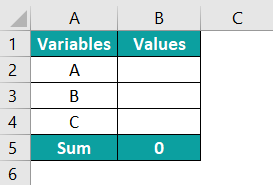We need to use the following steps to find the values of the three variables using solver in excel.

Step 2: Next, enter the SUM function in cell B5 to add the values of the three variables.

Step 3: Then, go to the Data tab.

Step 4: Click on the Solver option from the Analyze group.

Step 5: The Solver Parameters dialog box pops up.

Enter the data in the dialog box.

Click OK to see the values of the three variables.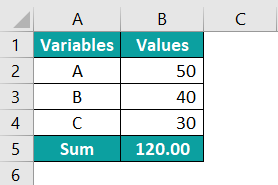Thus, we can obtain the values using solver in excel.

Why use Solver in Excel?

Solver in excel can be used to solve complex business models and to find the required variable values based on the constraints added.

Can Solver have multiple objectives in Excel?

Yes, the solver can be used to set multiple objectives in excel.